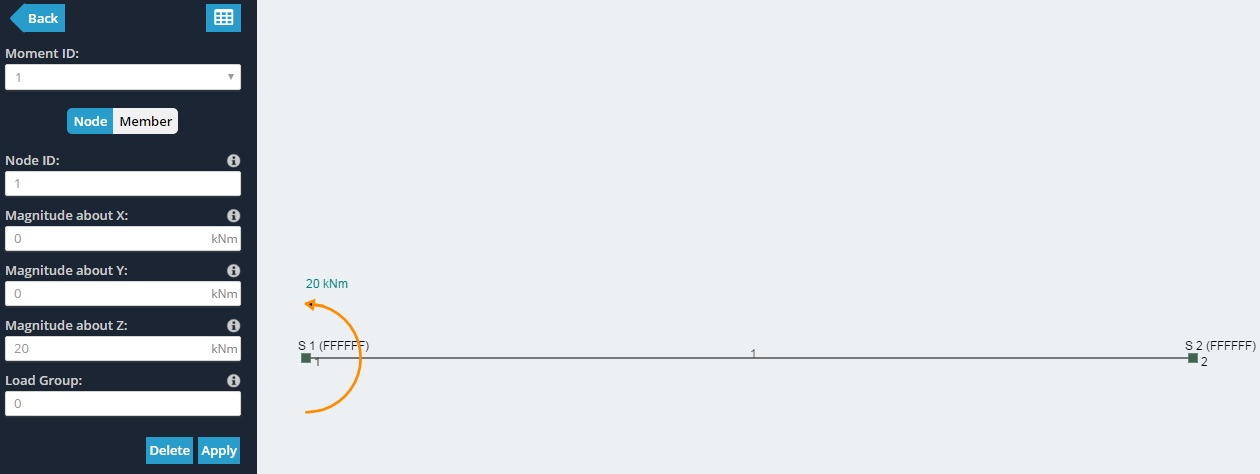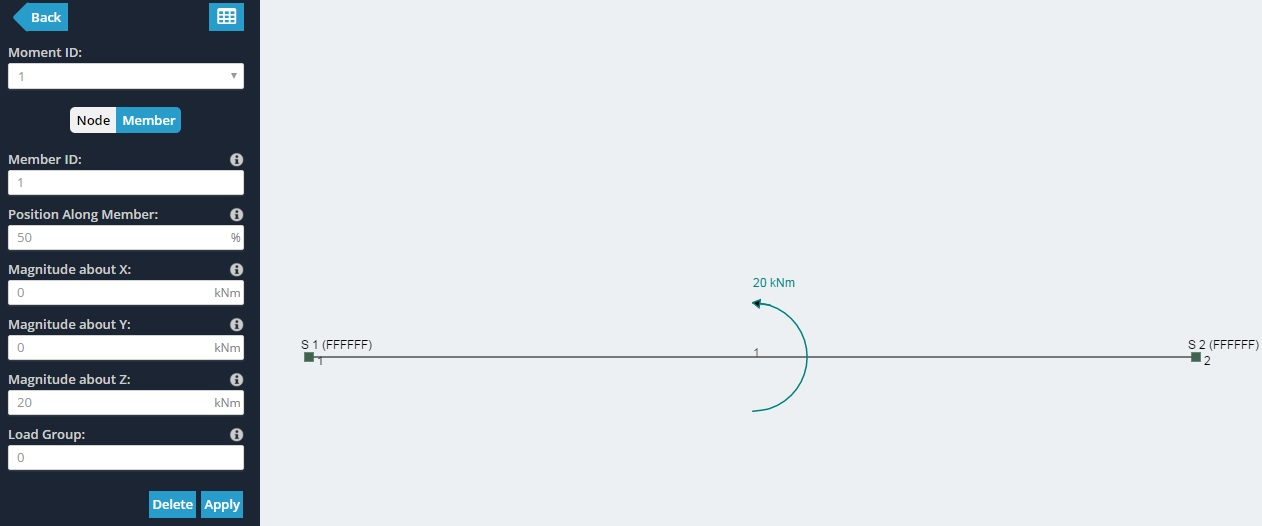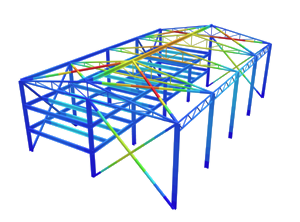SkyCiv Documentation

Your guide to SkyCiv software - tutorials, how-to guides and technical articles

# Moments

Moments are the product of force and distance relative to a point and induce rotational force. Moments can be applied to nodes or at a point along a member. In both cases, the location, magnitude, and sign direction need to be specified. Moments which are applied at an angle to the node or member can be specified by providing the X,Y,Z components of the moment.

Moments follow the sign convention of the “right hand” grip rule. The sign convention is dependent on which axis the moment is applied to:

• Positive (+): Counter-Clockwise
• Negative (-): Clockwise

## Moments applied to Nodes

To apply a point load on a node, simply specify values for:

• Node ID – The node where the moment is applied.

Here is an example of a 20kNm moment being applied to an end node looks like:## Moments applied to Members

To apply a point load on a member, simply specify values for:

• Member ID – The member where the point load is applied.
• Position Along Member – The % position (from node A t B) along a member where the force is to be applied.
• Magnitude about X – The magnitude of the force in the global X direction. Use negatives to specify the negative X direction.
• Magnitude about Y – The magnitude of the force in the global Y direction. Use negatives to specify the negative Y direction.
• Magnitude about Z – The magnitude of the force in the global Z direction. Use negatives to specify the negative Z direction.

Here is an example of a 20kNm moment being applied to the middle of a member looks like: